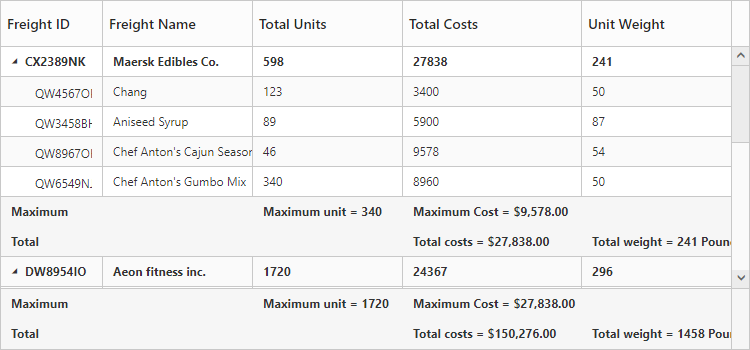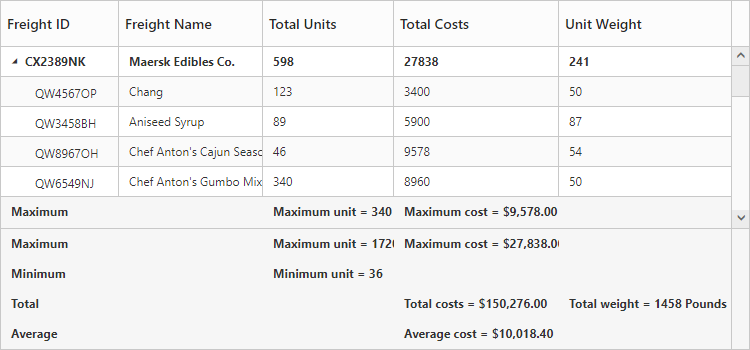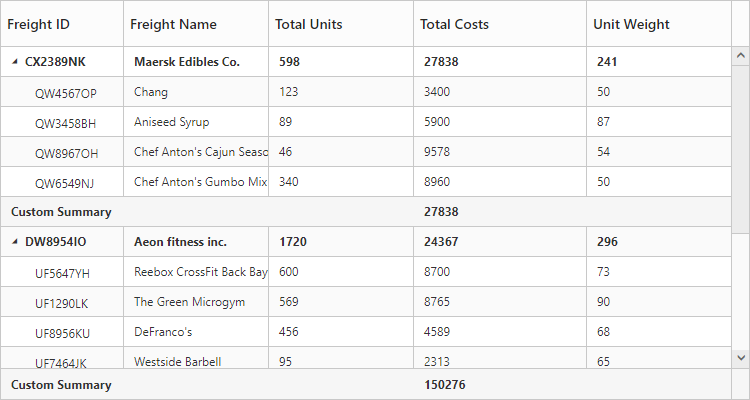# Summary Row

24 Sep 201812 minutes to read

Summary rows in TreeGrid is used to summarize every hierarchy with the set of predefined summary types using the column values.

• `e-summaryrows` - Using this property, user can define the summary rows in TreeGrid.
• `e-summaryrows.title` - Title for each summary row can be defined using this property.
• `e-summaryrows.summaryColumns` - Using this property, it is possible to defined the summary for specific columns alone in a summary row.
• `e-showsummaryrow` - This property is to make the summary row visible.
• `e-showtotalsummary` - This property is to make the total summary row visible which is the overall summary row displayed for all the rows in the TreeGrid content.

## Defining summary columns

The below code snippet explains defining a summary row in TreeGrid,

• JS
• ``````<body ng-controller="TreeGridCtrl">
<div id="TreeGridContainer" ej-treegrid
//...
e-summaryrows="summaryRows"
e-showsummaryrow="true"
e-showtotalsummary="true">
</div>
<script>
var summaryRows = [{
title: "Maximum",
summaryColumns: [
{
summaryType: ej.TreeGrid.SummaryType.Maximum,
dataMember: "TotalUnits",
displayColumn: "TotalUnits",
prefix: "Maximum unit = "
},
{
summaryType: ej.TreeGrid.SummaryType.Maximum,
dataMember: "TotalCosts",
displayColumn: "TotalCosts",
prefix: "Maximum Cost = ",
format: "{0:C}"
}
]
},
{
title: "Total",
summaryColumns: [
{
summaryType: ej.TreeGrid.SummaryType.Sum,
dataMember: "TotalCosts",
displayColumn: "TotalCosts",
prefix: "Total costs = ",
format: "{0:C}"
},
{
summaryType: ej.TreeGrid.SummaryType.Sum,
dataMember: "UnitWeight",
displayColumn: "UnitWeight",
prefix: "Total weight = ",
suffix: " Pounds"
}]
}
];
angular.module('listCtrl', ['ejangular'])
.controller('TreeGridCtrl', function(\$scope) {
//...
\$scope.summaryRows = summaryRows;
});
</script>
</body>``````

The below screenshot shows the output of above code example.## Customize height of total summary

Using `e-totalsummaryheight` property we can customize the height of the total summary container.
The below code example shows how to update the footer summary container height.

• JS
• ``````<body ng-controller="TreeGridCtrl">
<div id="TreeGridContainer" ej-treegrid
//...
e-totalsummaryheight=120
e-showtotalsummary="true">
</div>
</body>``````

The below screenshot shows the output of above code example.## Expand/collapse total summary row

We can expand/collapse the total summary rows in TreeGrid using following methods.

• Using Expander Icon
• Using Method

### Using Expander Icon

We can enable expander icon in total summary row by using `e-collapsibletotalsummary` property. By default expander icon will be rendered in first row of 0th column in total summary rows.
Please find the below code example to enable collapsible total summary row in TreeGrid.

• JS
• ``````<body ng-controller="TreeGridCtrl">
<div id="TreeGridContainer" ej-treegrid
//...
e-collapsibletotalsummary="true"
e-showtotalsummary="true">
</div>
</body>``````NOTE

We can also customize the expander icon column in total summary row by using `_summaryColumnIndex` property and `e-load` event.

### Using Method

Total summary rows in TreeGrid can be expanded/collapsed by using `expandCollapseTotalSummary` method.
Please find the code example to collapse the total summary rows below.

• HTML
• ``````<body ng-controller="TreeGridCtrl">
<button onclick="expandCollapse()">expandCollapse</button>
<div id="TreeGridContainer" ej-treegrid
//...
e-collapsibletotalsummary="true"
e-showtotalsummary="true">
</div>
</body>``````
• JS
• ``````function expandCollapse() {
var treeObj = \$("#TreeGridContainer").data("ejTreeGrid");
treeObj.expandCollapseTotalSummary(false);
}``````

## Custom Summary

Custom summary can be used to create summary values based on your required custom logic and calculations. To enable the custom summary, the summaryType should be set to ‘custom’ and the customSummaryValue property should be defined as function. After the custom calculation, the returned value will be displayed in the corresponding summary cell.

• HTML
• ``````<div ej-treegrid id="TreeGrid" e-summaryrows="summaryRows" e-showsummaryrow=true>
</div>``````
• JS
• ``````var summaryRows = [{
title: "Custom Summary",
summaryColumns: [{
summaryType: ej.TreeGrid.SummaryType.Custom,
customSummaryValue: currency,
displayColumn: "TotalCosts"
}]
}];
angular.module('listCtrl', ['ejangular'])
.controller('TreeGridCtrl', function(\$scope) {
//…
\$scope.summaryRows = summaryRows;
});``````

The output of the tree grid with custom summary value is as follows.